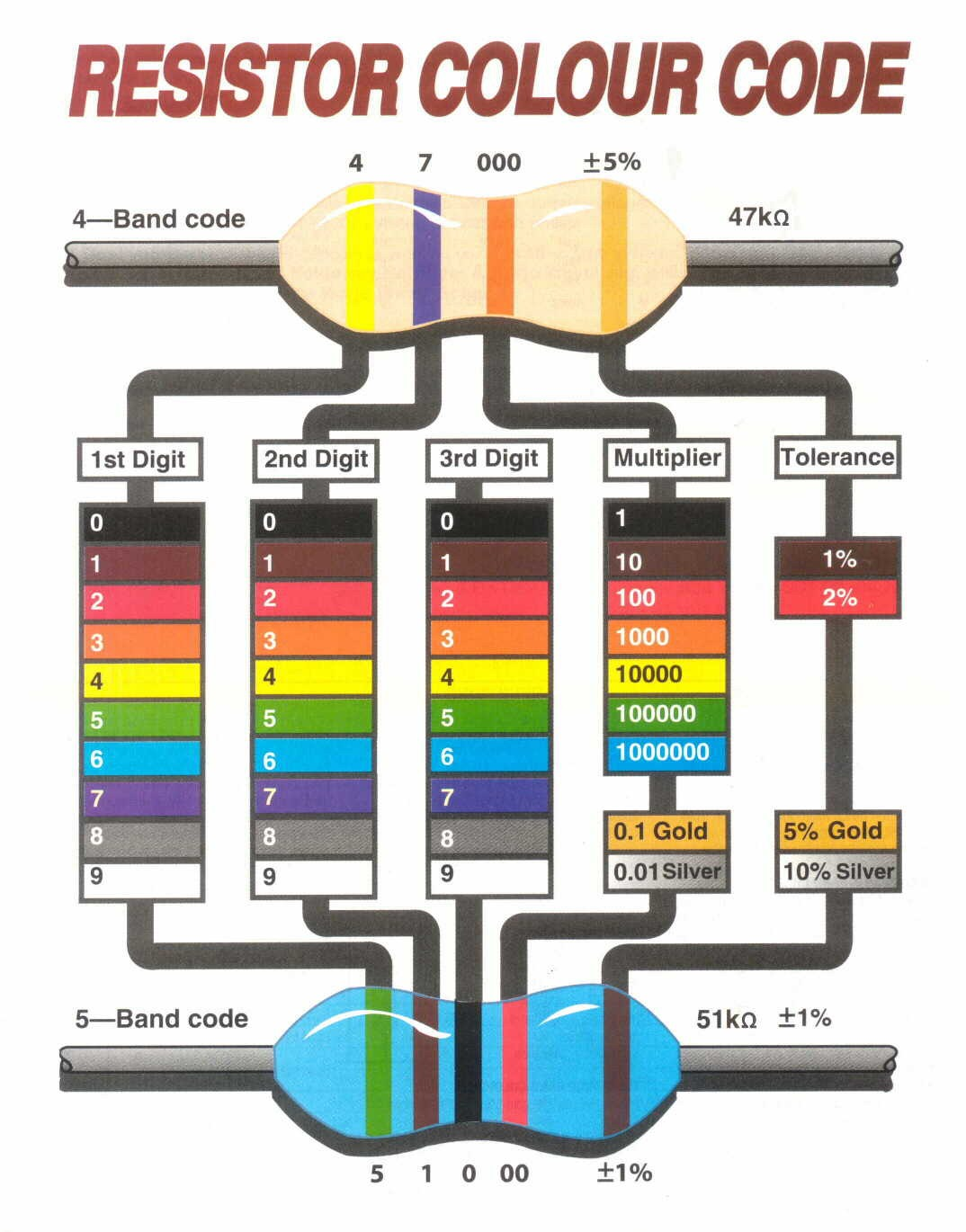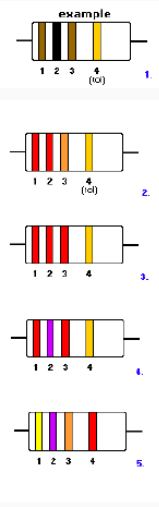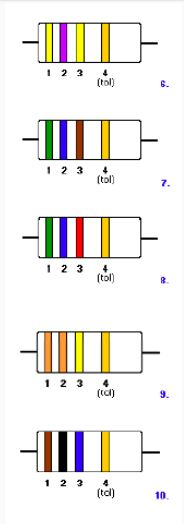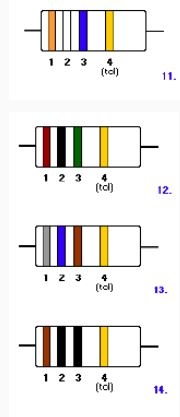# Resistors

Resistors resist the flow of electrons and protect some of the electrical components, like our LEDs.
Resistors can resist with different strengths. The way they are built will dictate how much they resist.

We can tell the resistance of a resistor by reading its 4 or 5 band code:In the first resistor above the bands are yellow, purple, orange and Gold.
1st band: 1st Digit (it's yellow so first digit is 4)
2nd band: 2nd Digit (it's purple, so second digit is 7)
3rd band: The multiplier (it's orange so multiply by 1000)
Last band: The tolerance (it's gold so tolerance is 5%).

This means that the first resistor resists with 47000 ohms at a tolerance of 5%.
The tolerance is the margin of error, so this resistor will resist within 5% of 47000 ohms.
Very expensive resistors have lower tolerances (like 1% or 2%).

It might be helpful to watch this short video:

Your task now is to complete this worksheet using the resistors below for each example.